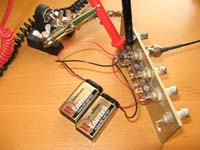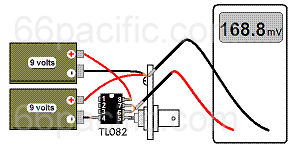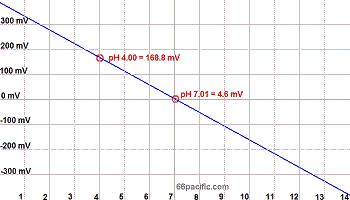# Building the Simplest Possible pH Meter

Build a digital pH meter that you can use instead of an expensive industrial ph meter or benchtop ph meter for a fraction of the cost.

### How the pH Meter Works

You can build a simple digital pH meter with just an inexpensive operational amplifier ("op amp") IC (a TL082 Dual JFET-input IC, about \$2.00), 2 batteries, a digital volt meter, and a pH probe (mine's a Pinpoint brand probe that I got from Amazon.com for about \$40).The TL082 (or any other operational amplifier with a high impedance input) works as a unity gain buffer between the high impedance of the pH probe and the digital multimeter.### Schematic Diagram of the pH Meter

Here's the simple schematic diagram of the pH meter circuit. Two 9-volt batteries power a high-input-impedance operational amplifier, such as a TL082. The pH probe of the meter is connected to the non-inverting input. The output voltage (V out), which is directly proportional to pH, is read with a voltmeter.

### View of the pH Meter Circuit

Here's a view of the entire pH meter circuit.The IC is mounted directly to a BNC panel connector.### Pictorial View of the pH Meter

This diagram shows the physical layout of the meter.A digital voltmeter is connected to ground and pins 6 and 7 of the  op amp, which are shorted together. In this circuit, pins 1, 2, and 3 of the IC are not used.

### Close-Up View

This pH meter circuit is so small and so simple that no circuit board is required. In this prototype, the IC is soldered directly to the BNC panel connector.### Calibration and Use of the pH Meter

In theory, a pH probe produces about 59 millivolts (mV) per pH unit, and at pH 7 (neutral pH) the probe produces 0 volts. Acid pHs produce negative voltages. Basic pHs produce positive pHs. For example, the system shown here reads +7.6 mV with the inputs shorted (due to the input offset error of the op amp). With the probe in pH 7.01 calibration buffer, the voltage is +4.6 mV. With pH 4.00 calibration buffer, the output voltage is 168.8 mV. This gives a range of 168.8 - 4.6 = 164.2 mV for 3.01 pH units, or 54.55 mV per pH unit.

If, for example, I measure 100 mV, the pH is 100 mV / 54.55 mV = 1.83 pH units deviation from 7.01, so I subtract 1.83 from 7.01 for a pH of 5.18. Creating a graph or a programming a calculator to do the arithmetic makes the process of determining pH simple, if not quite as simple as reading it off a  benchtop pH meter's display.

### Graphing the Results

Here's a graph of pH and voltage from the calibration of the setup described here.### pH Circuit for Arduino

Finally, and even simpler, if you are looking for a way to connect a pH probe directly to Arduino without building your own circuitry, you can buy a complete pH development kit for Arduino with a pH probe, calibration solutions, and pH-Stamp circuit for about \$100. The pH-Stamp, which you can also buy separately, is a complete pH monitoring system that enables you to accurately monitor pH without having to add any additional circuitry or components to your design. Communication with the pH-Stamp is done with 11 simple commands. It provides scientific-grade readings to any embedded system that has an RS232 connection interface (voltage swing 0-VCC, not +/- 12 volts).